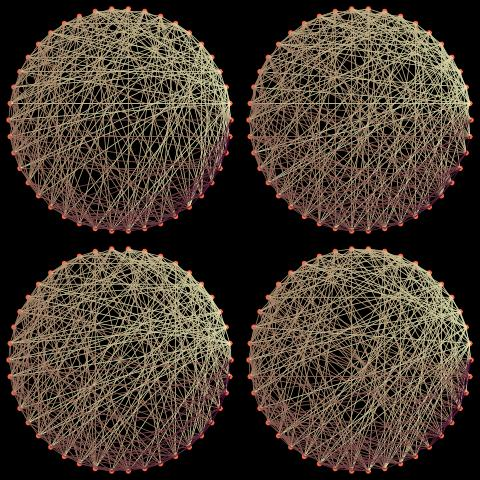Want to share your content on R-bloggers? click here if you have a blog, or here if you don't.In connection with Le Monde puzzle #46, I eventually managed to write an R program that generates graphs with a given number n of nodes and a given number k of edges leaving each of those nodes. (My early attempt was simply too myopic to achieve any level of success when n was larger than 10!) Here is the core of the R code:

```A=42 #number of nodes
L=13 #number of edges
ApL=A+L

if ((A*L)%%2==1){
print("impossible graph")
}else{
con=matrix(0,A,A)
diag(con)=A      #eliminate self-connection
suma=apply(con,1,sum)-A

while (min(suma)<L){

if (sum(suma<L)==1){ #bad news: no correspondence!
#go back:
con=aclrtr(con,L)
diag(con)=A
suma=apply(con,1,sum)-A

}else{

j=sample((1:A)[suma<L],1)
slots=(1:A)[con[j,]==0]  #remaining connections
if (length(slots)==1){
vali=slots
if (sum(con[vali,]>ApL-1)) vali=NULL
}else{
vali=slots[apply(con[slots,],1,sum)<ApL]
}

if (length(vali)==0){

con=aclrtr(con,L)
diag(con)=A
suma=apply(con,1,sum)-A

}else{

if (length(vali)==1){
k=vali
}else{
k=sample(slots[apply(con[slots,],1,sum)<ApL],1)
}
con[k,j]=con[j,k]=1
suma=apply(con,1,sum)-A
}}}}
```

and it uses a sort of annealed backward step to avoid simulating a complete new collection of neighbours when reaching culs-de-sac….

```aclrtr=function(con,L){
#removes a random number of links among the nodes with L links

A=dim(con)
ApL=A+L
while (max(apply(con,1,sum))==ApL){

don=sample(1:(L-1),1)
if (sum(apply(con,1,sum)==ApL)==1){
i=(1:A)[apply(con,1,sum)==ApL]
}else{
i=sample((1:A)[apply(con,1,sum)==ApL],1)
}
off=sample((1:A)[con[i,]==1],don)
con[i,off]=0
con[off,i]=0
}
con
}
```

There is nothing fancy or optimised about this code so I figure there are much better versions to be found elsewhere…

Ps-As noticed before, sample does not work on a set of length one, which is a bug in my opinion…. Instead, sample(4.5,1) returns a random permutation of (1,2,3,4).

> sample(4.5)
 4 3 1 2

Filed under: R, Statistics Tagged: edges, graphs, Le Monde, nodes, simulation